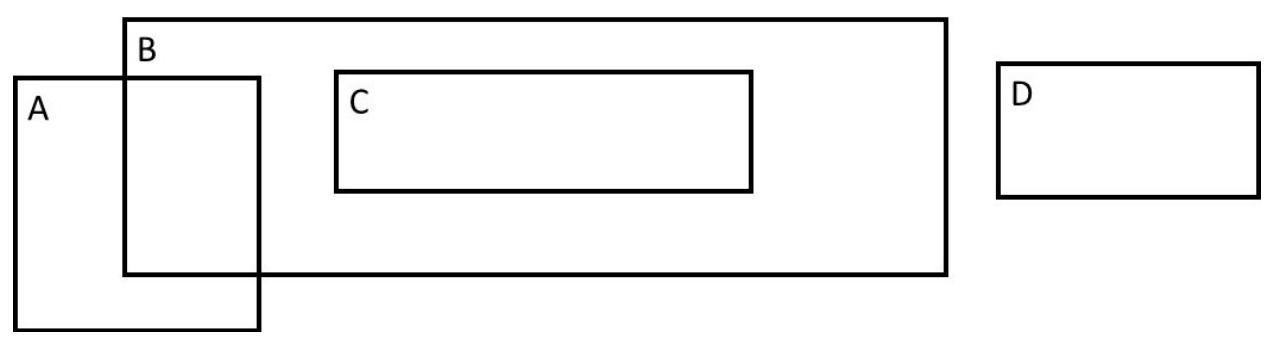시간 제한메모리 제한제출정답맞힌 사람정답 비율
2 초 512 MB22161473.684%

## 문제

You are given a set of n axis-aligned rectangles in a 2D plane. For this problem, two rectangles are considered to intersect if their boundaries contain any common points (in particular, two nesting rectangles don’t count as intersecting). Determine if some pair of rectangles intersect.In this example, only rectangles A and B intersect.

## 입력

Each test case will begin with a line with a single integer n (1 ≤ n ≤ 105), which is the number of rectangles.

Each of the next n lines will contain four space-separated integers:

x1 y1 x2 y2

(−109 ≤ x1, y1, x2, y2 ≤ 109, x1 < x2, y1 < y2), which describe a rectangle, where (x1, y1) is the lower left corner and (x2, y2) is the upper right corner. All x values will be distinct. All y values will be distinct.

## 출력

Output a single integer, which is 1 if some pair of rectangles intersect, 0 if no pair of rectangles intersect.

## 예제 입력 1

3
0 0 2 2
1 1 3 4
5 7 6 8


## 예제 출력 1

1


## 예제 입력 2

4
0 0 20 20
1 1 3 4
2 10 9 12
11 3 19 18


## 예제 출력 2

0